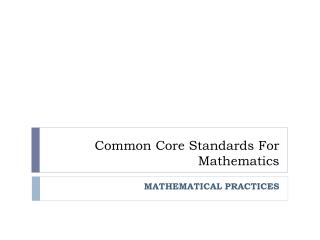Download PresentationCommon Core Standards For Mathematics# Common Core Standards For Mathematics - PowerPoint PPT Presentation

Download Presentation##### Common Core Standards For Mathematics

Download Policy: Content on the Website is provided to you AS IS for your information and personal use and may not be sold / licensed / shared on other websites without getting consent from its author. While downloading, if for some reason you are not able to download a presentation, the publisher may have deleted the file from their server.

- - - - - - - - - - - - - - - - - - - - - - - - - - - E N D - - - - - - - - - - - - - - - - - - - - - - - - - - -
##### Presentation Transcript

1. Common Core Standards ForMathematics MATHEMATICAL PRACTICES

2. FCUSD Implementation Plan (review) 2011-2012 Phase 1 Informational Introduction to Math Practices Using current math resources to teach Mathematical Practices ELA – Overview + Informational Text 2012-2013 Phase 2 Partial Implementation Elementary & Secondary Math – PD Math Practices and Understanding Grade Level Standards ELA – Informational Text + Writing 2013-2014 Phase 3 Partial Implementation Elementary Math - PD Math Practices & Grade Level Standards; Understanding Assessments Secondary Math - PD Math Practices begin transition to selected standards ELA - Informational Text + Writing + Collaborative Conversations; Understanding Assessment Elementary & Secondary Math – Full implementation ELA – Full implementation Assessments 2014-2015 Phase 4 Full Implementation

3. Standards for Mathematical Practice Two Types of Math Standards Within Common Core Describe varieties of expertise that educators should seek to develop in their students Identify processes and proficiencies that are hallmarks of mathematically proficient students K-8 grade level standards Remain the same throughout all grades K-12 9-12 standards organized by conceptual category (NOT by course)

4. Common Core Standards Overview Domain Standards Mathematical Practices

5. Standards for Mathematical Practice HABITS OF MIND

6. CCSS Mathematical Practices Standards for Mathematical Practice • 1. Make sense of problems and persevere in solving them • 2. Reason abstractly and quantitatively. • 3. Construct viable arguments and critique the reasoning of others. • 4. Model with mathematics. • 5. Use appropriate tools strategically. • 6. Attend to precision. • 7. Look for and make use of structure. • 8. Look for and express regularity in repeated reasoning.

7. PLAY

8. 1. MAKE SENSE OF PROBLEMS AND PERSEVERE IN SOLVING THEM. • Students need to develop a “puzzler’s disposition” • Give them a chance to puzzle things out using what they know • Remember: mysteries are fun but not if someone tells you the answer before you even try to solve it! • Too often students jump in to attempt a solution too fast. • They just want to “do” something to the numbers. • Modify questions so they ask for more thought and not only for a numeric “answer.”

9. Common type of fraction problem: What fraction of the bar is shaded blue? What kind of mathematical thinking was required? • Procedural • Definitional

10. Promoting Mathematical Thinking What fraction of the white strip is the pink strip?

11. What kind of mathematical PRACTICES did the fraction problems require? • Math Practice 1: Make sense of problems and persevere in solving them. • Math Practice 2: Reason abstractly and quantitatively. • Math Practice 3: Construct viable arguments and critique the reasoning of others. • Math Practice 5: Use appropriate tools strategically. • Math Practice 6: Attend to precision. • Much more attention than “mathematical reasoning” standards

12. Finding Mathematical Practices in our current program • Transitioning to Mathematical Practices with our current resources • Look at a problem-solving lesson in your TE • Tweak the lesson to make it more aligned to the mathematical practices. • What if you let the students work on the problem before you showed them each step to take?

13. Question from our book: What number do these base ten blocks represent?

14. Incorporating Math Practices Can you find a way to make 124 using only tens and ones? Can you find a different way? Find as many ways as you can to make 124 using hundreds, tens, and ones. If you think you have found all the ways, explain how you know your list is complete?

15. 3. Construct viable arguments and critique the reasoning of others. • Students talk about math and explain their thinking. • They construct arguments using concrete referents such as objects, drawings, diagrams, actions. • Students at all grades can listen or read the arguments of others, decide whether they make sense, and ask useful questions to clarify or improve the arguments. • One kind of task that naturally “pulls” children to explain is a “How many ways can you…” task.

16. Textbook activity • In your TE find a “Talk About It” exercise. • Work with a partner: if necessary, how can you make it a more mathematically rich discussion? • Mathematical Practices 3: • Construct viable arguments and critique the reasoning of others. “What are the factors for 12?” Find two numbers whose factors include 2, 3, 4, 6. How are the two numbers related?

17. Work to develop new “habits of mind” Give kids the background necessary to puzzle through problems Use the resources you have: tweak it here and there Lots of information on the internet Continuing professional development through district and Sacramento County Office of Education Putting it into Practice

18. For more information: • Common Core State Standards initiative: www.corestandards.org • California Common Core Standards: www.cde.ca.gov/ci/cc/ • Assessment: www.smarterbalance.org • Mathematical Practices: http://thinkmath.edc.org

19. Common Core State Standards • Exit Ticket • I learned… • I valued… • I’d like more information about… • Comments… Thank you!